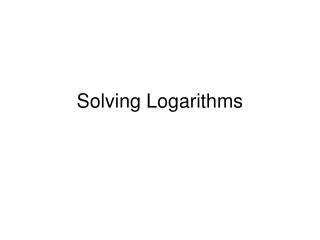# Solving Logarithms - PowerPoint PPT PresentationDownload PresentationSolving Logarithms

Solving LogarithmsDownload Presentation## Solving Logarithms

- - - - - - - - - - - - - - - - - - - - - - - - - - - E N D - - - - - - - - - - - - - - - - - - - - - - - - - - -
##### Presentation Transcript

1. Solving Logarithms

2. Solving for time (using logarithms) • To solve for time, you can get an approximation by using Excel. • To solve an exponential equation algebraically for time, you must use logarithms. • There are many properties associated with logarithms. We will focus on the following property: log ax = x * log a for a>0

3. A Petri dish contains 100 bacteria cells. The number of cells increases 5% Every minute. How long will it take for the number of cells in the dish to reach 3000? One way to solve this problem is to use excel. If you continue the chart, you will find that after 69 minutes, there were 2898 cells and at 70 minutes, there were 3043 cells. Obviously somewhere between minute 69 and 70, the number of cells reached 3000. However, using logarithms we can get an exact answer. Example Problem

4. A Petri dish contains 100 bacteria cells. The number of cells increases 5%Every minute. How long will it take for the number of cells in the dish toreach 3000? Start with : Y= P * (1 + r)X. Fill the variables that you know. To use logarithms, x must be your “unknown” quantity. The equation for this situation is: 3000 = 100 * (1+.05)X We need to solve for x: Step 1: divide both sides by 100 30 = (1+.05)X Step 2: take the log of both sides log 30 = log (1+.05)X Step 3: bring the x down in front log 30 = x * log(1+.05) Step 4:divide by log (1+.05) Enter the following into a cell in excel: =log(30)/log(1+.05) to get 69.71 (Of course you may use a calculator.) This tells us that at 69.71 minutes, there are 3000 cells.

5. Problem 2 Example If you \$100 deposited into a savings account grows at 3.4% compounded annually, how long will it take for your balance to double? Solving without logarithms: One way to solve this problem is to use excel. If you continue the chart, you will find that after 20 years, there will be \$195.17 in your account and after 21 years, there will be \$201.80 in your account. Obviously somewhere between years 20 and 21 the amount in your account will double from \$100 to \$200. However, using logarithms we can get an exact answer.

6. Problem 2 Example Solving with logarithms: Start with : Y= P * (1 + r)X. Fill the variables that you know. To use logarithms, x must be your “unknown” quantity. The equation for this situation is: 200=100*(1+.034)X We need to solve for x: Step 1: divide both sides by 100 2 = (1+.034)X Step 2: take the log of both sides log 2 = log(1+.034)X Step 3: bring the x down in front log 2 = x* log (1+.034) Step 4:divide by log (1+.034) Enter the following into a cell in excel: =log(2)/log(1+.034) to get 20.731 years (Of course you may use a calculator.) This tells us that at there will be \$200 in your account in 20.731 years or it means that will take 20.731 years for the money in your account to double.